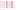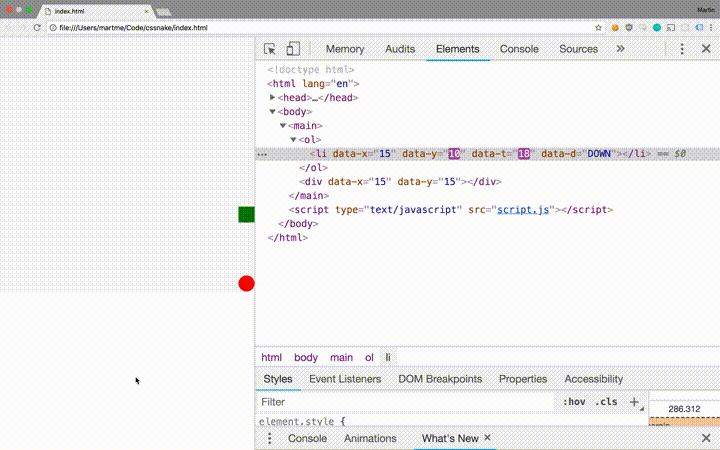# Snake in CSS

Published

Just because you can doesn't mean it's a good idea. But why not do it anyway?Simple implementations of a Snake game are rather forgiving - you only need to be able to draw moving squares on the screen and act upon keyboard input. CSS3 enable browser animations and HTML elements can be styled as squares. I wanted to leverage this and make a simple snake game with minimal use of JavaScript.

Source code available on github.

## Why?

Because inspecting the DOM while running the game is mesmerizing!Want to try? Go to martme.github.io/css-snake/.

## Implementation

The snake is an ordered element of squares starting with a head. Additional elements are added for each apple eaten by the head. Thus we can represented a snake as list items `<li>` in an ordered list `<ol>`. The apple is represented as a `<div>` element.

Elements are given a width and height using CSS, ensuring their square shape. Their position in a grid is denoted by `data-x` and `data-y` attributes on the HTML element. All elements have the CSS property `position: absolute` and CSS for corresponding `top` and `left` values are generated with SCSS as follows:

``````@for \$i from 0 to \$n {
[data-x="#{\$i}"] {
left: calc(#{\$i} * 100% / #{\$n});
}
[data-y="#{\$i}"] {
top: calc(#{\$i} * 100% / #{\$n});
}
}``````

Here the elements' parent has `position: relative` and a defined `width` and `height`. The variable `\$n` denotes the number of rows and columns in the grid. The size of the elements can be defined as follows:

``````\$n: 16;
li,
div {
width: calc(100% / #{\$n});
height: calc(100% / #{\$n});
position: absolute;
}``````

Movement is achieved using CSS animations. A move is completed in a single 'tick' thus ticks occur at an interval defined by the animation duration chosen in CSS. An element can move a distance equal to its size, either vertically or horizontaly, over the duration of a tick. This yields can be expressed using CSS.

``````@keyframes RIGHT {
0% {
transform: translateX(0);
}
100% {
transform: translateX(calc(100vh / #{\$n}));
}
}
@keyframes LEFT {
0% {
transform: translateX(0);
}
100% {
transform: translateX(calc(-100vh / #{\$n}));
}
}
@keyframes UP {
0% {
transform: translateX(0);
}
100% {
transform: translateY(calc(-100vh / #{\$n}));
}
}
@keyframes DOWN {
0% {
transform: translateX(0);
}
100% {
transform: translateY(calc(100vh / #{\$n}));
}
}``````

The duration of each animation 'tick' is denoted using the variable `\$t` and the direction is denoted by the `data-d` attribute of the HTML element. Referencing the keyframes, the pieces are given the ability to move with the following CSS.

``````\$t: 150ms;
li {
&[data-d="RIGHT"] {
animation: RIGHT #{\$t} linear infinite;
}
&[data-d="LEFT"] {
animation: LEFT #{\$t} linear infinite;
}
&[data-d="UP"] {
animation: UP #{\$t} linear infinite;
}
&[data-d="DOWN"] {
animation: DOWN #{\$t} linear infinite;
}
}``````

The animations alone are not sufficient to create fluid movement across the screen, as the element will jump back to start after the animation completes. Hence we need to update the coordinate attributes of elements following the completion of a tick. After each animation iteration, the event `animationiteration` is fired, which can be bound to custom behaviour in JavaScript. The following event handler adjusts the coordinate of the element according to the animated direction.

``````element.addEventListener("animationiteration", function(e) {
let x = +this.getAttribute("data-x")
let y = +this.getAttribute("data-y")

if (e.animationName === "RIGHT") x += 1
else if (e.animationName === "LEFT") x -= 1
else if (e.animationName === "DOWN") y += 1
else if (e.animationName === "UP") y -= 1

this.setAttribute("data-x", x)
this.setAttribute("data-y", y)
})``````

Since the `animationiteration` event is fiered independently for each of the elements constituting the snake, syncronization problems arise. This is solved using an additional HTML-attribute `data-t` to keep track of the number of ticks a given piece has completed. Thus if the tail is lagging behind, the next position can be computed to allow for the correct initial placement of the new tail.

If you made it all the way there, please remember that the only reason to do this is because the DOM looks cool if you inspect it while the snake is moving around the screen!